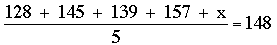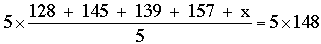SEARCH HOMEMath Central Quandaries & QueriesQuestion from Shelly, a student: (128 + 145 + 139 + 157 + x) divided by 5 = 148 matches the situation stated below: A person bowled four games and scored 128, 145, 139, and 157. Find x, the score the person would need to have in the fifth game to average 148 for all five games. How do I solve this and how am I supposed to add x like it says in the equation?Hi Shelly,

What you need to do is manipulate the equation to end up with an equation of the form

x = some number.

When you manipulate the equation you need to make sure that the two sides remain equal. The operation that do this are to add the same amount to each side or multiply each side by the same amount. With your equationI would first multiply each side by 5.or

128 + 145 + 139 + 157 + x = 740.

128 + 145 + 139 + 157 = 569 so I would next add -569 to each side.

When you find x make sure that you verify that the average score on the 5 games is 148.

PennyMath Central is supported by the University of Regina and The Pacific Institute for the Mathematical Sciences.